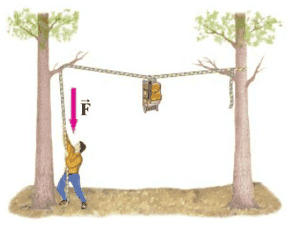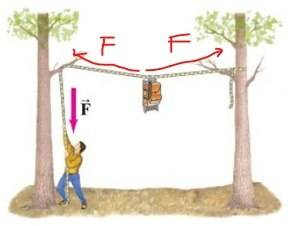# Static Equilibrium Problem (concept question)

Sunwoo Bae
Homework Statement:
The two trees shown in the figure are 6.6 m apart. A back-packer is trying to lift his pack out of the reach of bears. Calculate the magnitude of the force F that he must exert downward to hold a 19-kg backpack so that the rope sags at its midpoint by (a) 1.5 m, (b) 0.15 m.
Relevant Equations:
the sum of forces = 0 in static equilibrium(figure)

I was able to solve the problem and get the right answer. However, I solved it more with intuition, and I do not get how the force of the person pulling down on the rope makes the force to the left of the backpack equal to force to the right of the backpack.Homework Helper
force to the left of the backpack equal to force to the right
What would happen if this were not the case ?

•Lnewqban
I was able to solve the problem and get the right answer. However, I solved it more with intuition, and I do not get how the force of the person pulling down on the rope makes the force to the left of the backpack equal to force to the right of the backpack.

View attachment 260882

There are a few assumptions that need to be made for both forces on the backpack to be of magnitude ##F##; The contact at the branch needs to be frictionless and the rope needs to be massless.

Homework Helper
Gold Member
2022 Award
There are a few assumptions that need to be made for both forces on the backpack to be of magnitude ##F##; The contact at the branch needs to be frictionless and the rope needs to be massless.
But the OP's question is about the tensions in the rope either side of the backpack being equal. That does not require those assumptions, only that the pack is at the midpoint (which is not stated) and that the tree branches are at the same height.

•etotheipi
But the OP's question is about the tensions in the rope either side of the backpack being equal. That does not require those assumptions, only that the pack is at the midpoint (which is not stated) and that the tree branches are at the same height.

Right, it's just OP also mentioned the force pulling down on the rope. Indeed, the force on the left and right will be equal even if the two conditions I gave are not met. Sorry! If anything, it's decent pub quiz trivia... ish.

Last edited by a moderator:
Homework Helper
Gold Member
... I do not get how the force of the person pulling down on the rope makes the force to the left of the backpack equal to force to the right of the backpack.
Consider these three cases and discuss with us what your intuition tells you about the forces within the rope:
1) Rather than a person pulling down, the left end of the rope is anchored to the branch, just like the right end is (backpack is located midway).

2) There is no backpack and the person still pulls down very strongly.

3) Rather than only one person pulling down, the right end of the rope goes around a branch and is pulled down by a second person with similar amount of force (backpack is located midway).